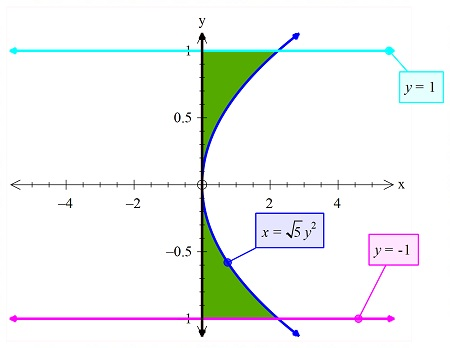# Find the volume of the solid of revolution. The region bounded by x = sqrt(5) y^2, x = 0, y = -1,...

## Question:

Find the volume of the solid of revolution.

The region bounded by {eq}\; x = \sqrt{5} y^2, \; x = 0, \; y = -1, \; y = 1 \; {/eq} revolved about the {eq}y {/eq}-axis.

## Volumes:

When computing the volume of revolution, we need to check the graph and the bounded region, along with the axis of rotation. This is done so as to we can decide on which method type can be used to find the volume. In the given problem we will use the disk method.

## Answer and Explanation:

Let use the graph to find the volume of revolution.

So the graphs of the common region is given below:Now since the graph is...

See full answer below.

Become a Study.com member to unlock this answer! Create your account

#### Learn more about this topic:How to Find Volumes of Revolution With Integration

from Math 104: Calculus

Chapter 14 / Lesson 5
11K